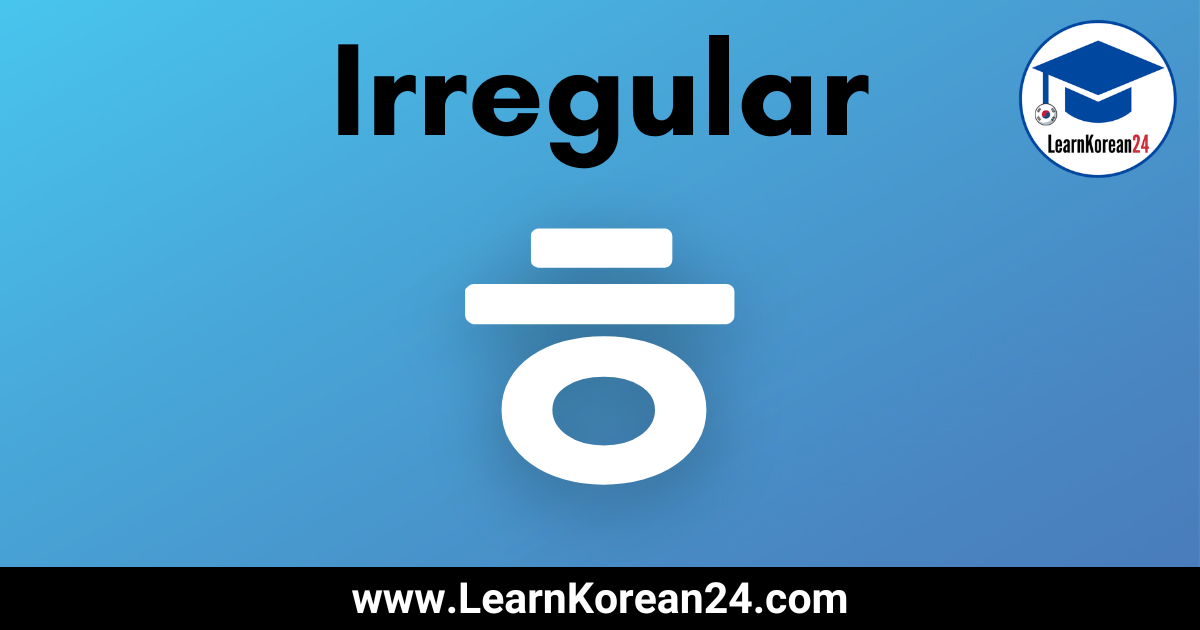# Irregular Korean Verbs With ㅎ (ㅎ 불규칙)In this lesson, you will learn about ㅎ irregular verbs (ㅎ 불규칙). In Korean, most verbs that end in ㅎ are regular verbs, and as such, they are conjugated according to the regular conjugation rules. However, there are some verbs/adjectives which end in ㅎ which are irregular.

Before we look at the list of irregular verbs with ㅎ and how to conjugate these irregular verbs, it is important to note that ㅎ irregular verbs are all ‘descriptive verbs’, or to be more accurate, they are adjectives.

When you look up an adjective in a Korean dictionary, it is listed in its basic verb form (e.g. 노랗다 = to be yellow). This is why many Korean textbooks refer to adjectives as ‘descriptive verbs’. In this lesson, we will simply refer to them as irregular ㅎ verbs, but please remember they are actually ‘descriptive verbs’/adjectives.

Below you will learn which verbs (adjectives) ending in ㅎ are irregular, and you will learn how to conjugate irregular ㅎ verbs in Korean.

## ㅎIrregular Verbs (ㅎ 불규칙)

First, to help you learn which Korean verbs/adjectives ending in ㅎ are irregular, here is a list of irregular ㅎ verbs/adjectives in Korean.

### ㅎ Irregular Verbs List

• 노랗다 = to be yellow
• 빨갛다 = to be red
• 파랗다 = to be blue
• 하얗다 = to be white
• 까맣다 = to be black
• 그렇다 = to be like that
• 저렇다 = to be like that
• 이렇다 = to be like this
• 어떻다 = to be such
• 뿌옇다 = to be hazy

## How To Conjugate ㅎ Irregular Verbs (ㅎ 불규칙)

Conjugating irregular verbs (adjectives) is a little tricky compared to the other irregular verb forms. When conjugating regular verbs that end in the verb stem doesn’t change. For example, 좋다 (to be good) + 아요 (present tense ending) becomes 좋아요. However, when conjugating irregular verbs that end in , the verb stem does change sometimes.

The first thing to know about conjugating ㅎ irregular verbs is that ㅎ irregular verbs only behave irregularly when attaching an ending that starts in a vowel. When attaching an ending that starts in a consonant, an irregular ㅎ verb is conjugated just the same as regular verbs.

Let’s take a look at how to conjugate irregular verbs (adjectives) with endings that start with a vowel. The particular conjugation rule to follow depends on which ending you are attaching to the irregular verb.

### Endings Starting With 아/어

When attaching endings which start with 아/어 (for example 아요/어요, 아서/어서,았어요/었어요) to a ㅎ irregular verb, the first thing you must do is drop ㅎ from the verb stem. For example, if you drop the from the descriptive verb 빨갛다 (to be red) it becomes 빨가.

Next, look at the last letter in the verb stem and if this letter is or , then you must change 아/어 to . If the last letter is or , then you must change 야/여 to or (야 changes to 얘 and 여 changes to 예).

So, in the above example, after removing from 빨갛다 you are left with the stem 빨가. 빨가 ends in and so this changes to .

빨갛다 + 아요 = 빨개요
빨갛다 + 아서 = 빨개서
빨갛다 + 았어요 = 빨갰어요

Here’s another example, this time with 하얗다 (to be white). Again, first, we must drop from the verb stem and so you are left with 하야. As you can see, 하야 ends with . So, when attaching an ending which starts with 아/어 we must change to .

하얗다 + 아요 = 하얘요
하얗다 + 아서 = 하얘서
하얗다 +았어요 = 하얬어요

### Endings Starting With (으)

When attaching endings which start with ~(으), (for example ~(으)면, ~(으)니까, ~(으)ㄹ 거예요), to irregular verbs, and are dropped. Let’s look at some examples with 그렇다 (to be like that).

그렇다 + ~(으)면 = 그러면
그렇다 + ~(으)니까 = 그러니까
그렇다 + ~(으)ㄹ 거예요 = 그럴 거예요

## ㅎ Irregular Conjugation Chart

To help you learn how to conjugate irregular ㅎ verbs, here is a printable ㅎ irregular conjugation chart.

## More Korean Irregular Verb Lessons

There are seven types of irregular verbs in Korean. For more lessons on Korean Irregular verbs, click on the links below.